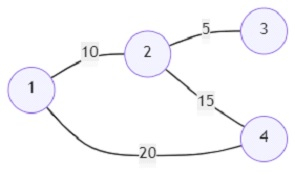# Program to find out the path between two vertices in a graph that has the minimum penalty (Python)

Suppose we are given an undirected, weighted graph and are asked to find out the path with the minimum possible penalty from node a to node b. The penalty of a path is the bitwise OR of the weights of all the edges in the path. So, we must find out such a 'minimum penalty' path, and if there exists no path between the two nodes, we return -1.

So, if the input is likestart (s) = 1, end (e) = 3; then the output will be 15.

There exist two paths between vertices 1 and 3. The optimal path is 1->2->3, the cost of the path is (10 OR 5) = 15.

To solve this, we will follow these steps −

• Define a function helper() . This will take G, s, e
• v := a new set
• c := a new list of size n initialized with value infinity
• heap := a new heap containing pair (0, s)
• while size of heap > 0, do
• cst := pop the smallest item from heap
• cur := pop the smallest item from heap
• c[cur] := minimum of (cst, c[cur])
• if (cst, cur) is present in v, then
• go for the next iteration
• if cur is same as e, then
• return c[cur]
• add pair (cst, cur) to v
• for each neighbor, n_cost in G[cur], do
• push values ((n_cost OR cst), neighbor) to heap
• return c[e]
• G := [a new list containing n + 1 emoty lists]
• for each item in edges, do
• u := item
• v := item
• w := item
• insert pair (v, w) at the end of G[u]
• insert pair (u, w) at the end of G[v]
• ans := helper(G, s, e)
• return -1 if ans is same as inf otherwise return ans

## Example

Let us see the following implementation to get better understanding −

import heapq
from math import inf

def helper(G, s, e):
v = set()
c = [inf] * len(G)
heap = [(0, s)]
while len(heap) > 0:
cst, cur = heapq.heappop(heap)
c[cur] = min(cst, c[cur])
if (cst, cur) in v:
continue
if cur == e:
return c[cur]
for neighbor, n_cost in G[cur]:
heapq.heappush(heap, (n_cost | cst, neighbor))
return c[e]

def solve(n, edges, s, e):
G = [[] for _ in range(n + 1)]
for item in edges:
u, v, w = map(int, item)
G[u].append((v, w))
G[v].append((u, w))
ans = helper(G, s, e)
return -1 if ans == inf else ans

print(solve(4, [(1, 2, 10), (2, 3, 5), (2, 4, 15), (1, 4, 20)], 1, 3))

## Input

4, [(1, 2, 10), (2, 3, 5), (2, 4, 15), (1, 4, 20)], 1, 3

## Output

15